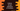# How to accept sequence of numbers in python and find out the averageThis tutorial will show you how to accept a sequence of numbers in python. For example, if you want to read a sequence of numbers to find out the average, maximum or minimum value.

In this post, we will write one python program that will take a sequence of numbers from the user and find out the average.

## How to get a list of numbers as input in python :

There is no straight-forward way to accept a sequence of numbers from the user. Instead, we can use one loop or we can accept all numbers in one go as comma separated values.

In case of a loop, we will ask the user to enter a number repeatedly and in case of comma separated values, we can read and split the values.

## Method 1: By using a loop :

With this method, we will take the total count of numbers first and read them one by one using a loop as like below :

``````num_list = []
total = int(input("Enter total count of numbers :"))

for i in range(0, total):
num_list.append(int(input("Enter number for index "+str(i)+" : ")))

print("Average : ", sum(num_list)/len(num_list))``````

Sample output :

``````Enter total count of numbers :3
Enter number for index 0 : 1
Enter number for index 1 : 2
Enter number for index 2 : 3
Average :  2.0

Enter total count of numbers :4
Enter number for index 0 : 1
Enter number for index 1 : 3
Enter number for index 2 : 5
Enter number for index 3 : 9
Average :  4.5``````

## Method 2: Reading all numbers in one go:

Another way to solve it is by taking the numbers as comma separated values. If we read them as comma separated values, we can split them and read them easily :

``````num_list = list(int(num) for num in input(
"Enter the numbers separated by comma :").strip().split(','))
print(sum(num_list)/len(num_list))``````

What we are doing here :

• Reading all numbers separated by comma
• Removing the extra spaces using stripe()
• splitting the values using split
• Using a for loop, it is converting all values to integer and creates a list

Sample output :

``````Enter the numbers separated by comma :1,2,3,4,5,6
3.5``````